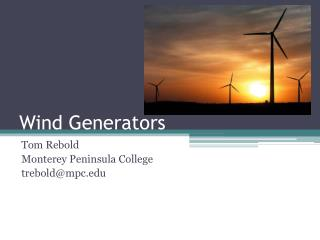DownloadDownload PresentationWind Generators

# Wind Generators

Télécharger la présentation## Wind Generators

- - - - - - - - - - - - - - - - - - - - - - - - - - - E N D - - - - - - - - - - - - - - - - - - - - - - - - - - -
##### Presentation Transcript

1. Wind Generators Tom Rebold Monterey Peninsula College trebold@mpc.edu

2. Need to know… • Basic Electricity Measurements • Power Calculations • Power in wind, electricity, and movement of water • Wind Tower Design (engineering statics) • Wind generator blade design (aerodynamics) • Water Pump Design (hydraulics)

3. Electrity: Three Main Invisible Quantities • Voltage, V, Volts • Provides the “push” • Current, I, Amperes (Amps) • Flow of Electrons • Amount of Current is dependent on Voltage and Resistance • Resistance, R, Ohms (W) • Limits the amount of current

4. Safe Levels • Voltage: 30 V • Voltages inside a computer do not exceed 12 V, except at the power supply and power switch, which are at 120 V.Be careful in these areas! • Current: 5 mA (0.005 Amperes)

5. Voltage Can Be Provided From… • A battery

6. Voltage Can Be Provided From… • A generator

7. Current • …is simply the flow of electrons • Direction depends on convention • Electron flow is from (-) to (+) (flow of electrons) • Conventional flow is from (+) to (-) (hole flow)

8. Resistors – Basic Specs • Can be rated by… • Resistance (Ohms, W) • Tolerance (% of nominal value) • Power Rating (Watts) • Schematic Symbol…

9. Resistors – Types • Fixed • Variable (Potentiometer, Rheostat)

10. Resistors – Color Code

11. 5% (gold) 3 3 102 Resistors –Colour Code Example • 1st band: orange = 3 • 2nd band: orange = 3 • 3rd band: red = 2 (i.e. 102) • 4th band: gold = 5% 33 x 102 = 3300 W = 3.3 k W

12. Resistors – Typical Power Ratings

13. Ohm’s Law “Current (I) is proportional to Voltage (V) and inversely proportional to Resistance (R)” UNITS: 1 Volt across 1 Ohm produces 1 Amp of current

14. How Many Volts across Resistor? 300 W 2A

15. Ohm’s Law, Including Power Reproduced by permission of Tony van Roon, 2002http://www.uoguelph.ca/~antoon

16. How Many Watts dissipated by Resistor? 300 W 2A P=I2R

17. How Many Watts dissipated by Resistor? 1.5 W I 6V P=I2R = V2/R = IV

18. For your Wind generator, same calculations apply Water Pump To find the power delivered to our pump, we will have to measure V and I I Wind Generator P=I2R = V2/R = IV

19. Complete System Drawing 10 ft wire Pump Fuse

20. Wind and Hydro Power Calculations • How much power is in the wind? • How much power does it take to pump a liter of water 2 m uphill in 5 minutes? • How can we evaluate how effective our wind system is at pumping water?

21. Engineering a Power Conversion System Wind Electrical Pumping Power Power Power Each conversion results in a loss of power 50 W 5 W 1 W This system has an overall efficiency of 1/50 or 2% In subsequent designs, engineers would try to improve the efficiency

22. The Power in the Wind • From basic physics we have “Kinetic Energy” • K = ½ M V2 • K = energy in Joules (or N-m, or even KW-hr) • M = mass, kg • V = velocity of object, m/s • In the context of wind, we can determine the mass of the cylinder of air that hits our generator in a certain time interval. • Power is the amount of Kinetic Energy we capture divided by the time we are gathering it. ( Watts)

23. Hydraulic Power • In order to pump a mass m kg of water h meters uphill, we require an amount of energy equal to • E = m g h (Joules) where g = 9.8 m/s2 • To pump uphill in T seconds, would require a power of • P = E/T (Watts) • Suppose m = 1 kg, d = 2 m, T = 60 s • P = 1 x 9.8 x 2 / 60 = .3 W

24. Tower Design • In ENGR 8 You can determinetension in cables! • Keep tower simple. 2x4 with 4 cords attached to stakes • Mount motor using steel bands and screws